GMAT Math : DSQ: Calculating the area of an acute / obtuse triangle

Example Questions

Example Question #1 : Dsq: Calculating The Area Of An Acute / Obtuse Triangle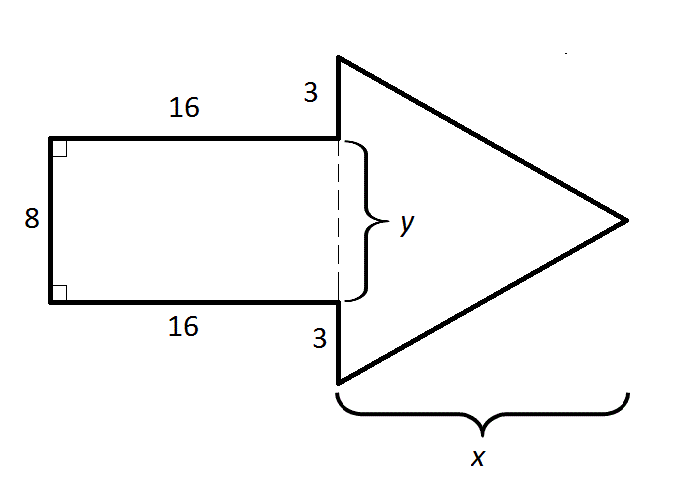Note: Figure NOT drawn to scale.

What is the area of the above arrow?

Statement 1: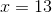Statement 2: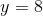Statement 1 ALONE is sufficient to answer the question, but Statement 2 ALONE is NOT sufficient to answer the question.

Statement 2 ALONE is sufficient to answer the question, but Statement 1 ALONE is NOT sufficient to answer the question.

BOTH statements TOGETHER are insufficient to answer the question.

EITHER statement ALONE is sufficient to answer the question.

BOTH statements TOGETHER are sufficient to answer the question, but NEITHER statement ALONE is sufficient to answer the question.

Statement 1 ALONE is sufficient to answer the question, but Statement 2 ALONE is NOT sufficient to answer the question.

Explanation:

It can already be ascertained from the figure that, since the left portion is a rectangle, so Statement 2 is redundant.

We can already calculate the area of the rectangular portion of the arrow: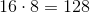All this is left is to calculate the area of the triangular portion. If we know Statement 1, we can take half the product of the height, which is 13, and the base, which is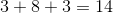: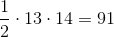Add these numbers to get the area of the arrow: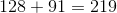Example Question #2 : Dsq: Calculating The Area Of An Acute / Obtuse Triangle

Two of the vertices of a triangle on the coordinate plane are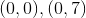. What is its area?

Statement 1: The-coordinate of the third vertex is 8.

Statement 2: The-coordinate of the third vertex is 5.

BOTH statements TOGETHER are insufficient to answer the question.

Statement 2 ALONE is sufficient to answer the question, but Statement 1 ALONE is NOT sufficient to answer the question.

EITHER statement ALONE is sufficient to answer the question.

Statement 1 ALONE is sufficient to answer the question, but Statement 2 ALONE is NOT sufficient to answer the question.

BOTH statements TOGETHER are sufficient to answer the question, but NEITHER statement ALONE is sufficient to answer the question.

Statement 1 ALONE is sufficient to answer the question, but Statement 2 ALONE is NOT sufficient to answer the question.

Explanation:

The triangle has as its base a vertical line of length 7, so the height of the triangle would be the perpendicular - which in this case is horizontal - distance from that base. Since the base is part of the-axis, this distance is the absolute value of the-coordinate, which is ony given by Statement 1. Statement 2 is irrelevant.

This is illustrated by this diagram: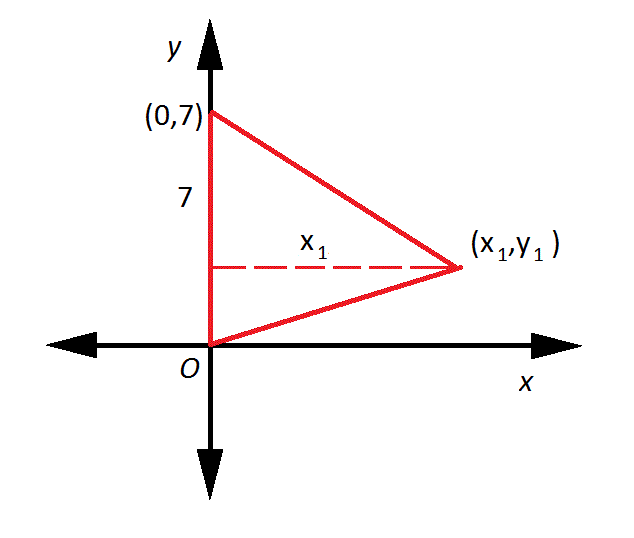Example Question #3 : Dsq: Calculating The Area Of An Acute / Obtuse Triangle

What is the area of a triangle on the coordinate plane with two of its vertices at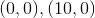?

Statement 1: The-coordinate of its third vertex is 6.

Statement 2: The-coordinate of its third vertex is 8.

BOTH statements TOGETHER are sufficient to answer the question, but NEITHER statement ALONE is sufficient to answer the question.

Statement 1 ALONE is sufficient to answer the question, but Statement 2 ALONE is NOT sufficient to answer the question.

EITHER statement ALONE is sufficient to answer the question.

Statement 2 ALONE is sufficient to answer the question, but Statement 1 ALONE is NOT sufficient to answer the question.

BOTH statements TOGETHER are insufficient to answer the question.

Statement 2 ALONE is sufficient to answer the question, but Statement 1 ALONE is NOT sufficient to answer the question.

Explanation:

The area of a triangle is half the product of its base and its height.

The triangle has as its base a horizontal line of length 10, so the height of the triangle would be the perpendicular - which in this case is vertical - distance from the third vertex to that base. Since the base is part of the-axis, this height is the absolute value of the-coordinate, which is only given by Statement 2. Statement 1 turns out to be irrelevant.

This is illustrated by this diagram: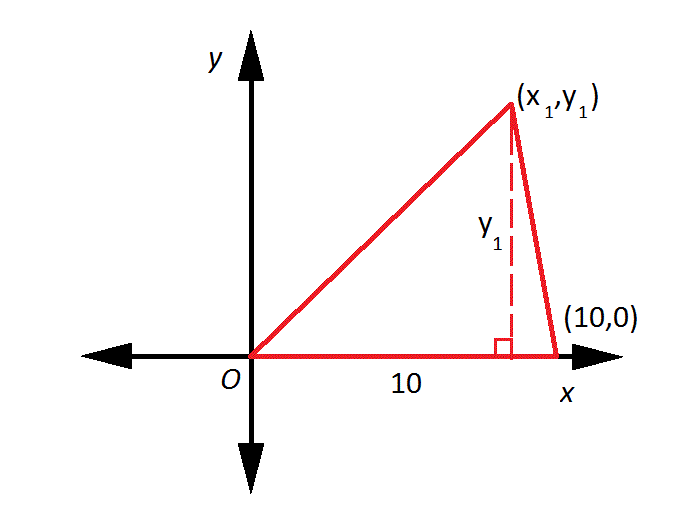Tired of practice problems?

Try live online GMAT prep today.